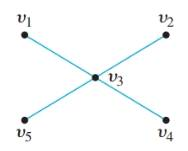Chapter 10.1, Problem 9ES### Discrete Mathematics With Applicat...

5th Edition
EPP + 1 other
ISBN: 9781337694193

#### Solutions

Chapter
Section### Discrete Mathematics With Applicat...

5th Edition
EPP + 1 other
ISBN: 9781337694193
Textbook Problem
4 views

# Each of (a)—(c) describes a graph. In each case answer yes, no, or not necessarily to this question: Does the graph have an Euler circuit? Justify your answers. a. G is a connected graph with five vertices of degrees 2, 2, 3, 3, and 4. b. G is a connected graph with five vertices of degrees 2, 2, 4, 4, and 6. c. G is a graph with five vertices of degrees 2, 2, 4. 4, and 6.

To determine

(a)

Does the graph have an Euler circuit, when G is a connected graph with five vertices of degrees 2, 2, 3, 3, and 4? Justify your answers.Explanation

Given information:

G is a connected graph with five vertices of degrees 2, 2, 3, 3, and 4.

Calculation:

An Euler circuit is a circuit that contains every edge of the graph.

A connected graph has an Euler circuit if and only if each of the vertices has an even degree...

To determine

(b)

Does the graph have an Euler circuit when G is a connected graph with five vertices of degrees 2, 2, 4, 4, and 6? Justify your answers.

To determine

(c)

Does the graph have an Euler circuit when G is a graph with five vertices of degrees 2, 2, 4, 4, and 6? Justify your answers.

### Still sussing out bartleby?

Check out a sample textbook solution.

See a sample solution

#### The Solution to Your Study Problems

Bartleby provides explanations to thousands of textbook problems written by our experts, many with advanced degrees!

Get Started